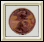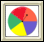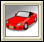# How Likely Is It?HOW LIKELY IS IT?

IXL Math

BBC Bitesize Math Probability ActivityCoin Tossing – Explore probability concepts by simulating repeated coin tosses.Hamlet Happens – Verify that rare events happen by drawing letters from a box.Spinners – Work with spinners to learn about numbers and probabilities.Stick or Switch – Investigate probabilities of sticking with a decision, or switching.Data Analysis & Probability Deal or No DealThe rules are simple. Choose a briefcase. Then as each round progresses, you must either stay with your original briefcase choice or make a "deal" with the bank to accept its cash offer in exchange for whatever dollar amount is in your chosen case.Data Analysis & Probability Guess the Number -1,000 to 1,000Guess a number from -1,000 to 1,000. What is the best strategy?Data Analysis & Probability Guess the Number 0 to 100Guess a number from 0 to 100. What is the best strategy?Data Analysis & Probability Probability - StarfishIt's a beautiful day at the beach. Let's learn about probability with starfish.Adjustable Spinner Creating a Spinner to Examine Experimental and Theoretical OutcomesFire Simulating the Spread of a Wildfire Using Probability
Create a game spinner with variable sized sectors to look at experimental and theoretical probabilities. Parameters: Sizes of sectors, number of sectors, number of trials.

Experiment with probability using a fixed size section spinner, a variable section spinner, two regular 6-sided dice or customized dice.

In this applet you can adjust the parameters on two Gaussian curves to determine if there is a possibility of a difference between the two means.

Create a game spinner with one to twelve sectors in order to look at experimental and theoretical probabilities. Parameters: Number of sectors, number of trials.
INSERT HERE

How Likely Is It?
(University Of Michigan)

Concept with Explanation
Selected Homework from ACE

The unit How Likely Is It? was created to help students:

• Understand that probabilities are useful for predicting what will happen over the long run

• Understand the concepts of equally likely and not equally likely;

• Understand that a game of chance is fair only if each player has the same chance of winning, not just a possible chance of winning;

• Understand that there are two ways to build probability models: by gathering data from experiments (experimental probability) and by analyzing the possible equally likely outcomes (theoretical probability);

• Understand that experimental probabilities are better estimates of theoretical probabilities when they are based on larger numbers of trials;

• Develop strategies for finding both experimental and theoretical probabilities; and

• Critically interpret statements of probability to make decisions or answer questions.